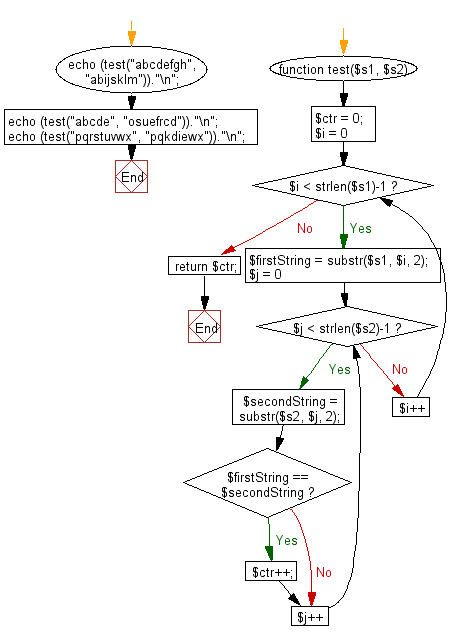﻿ PHP Exercises: Compare two given strings and return the number of the positions where they contain the same length 2 substring - w3resource# PHP Exercises: Compare two given strings and return the number of the positions where they contain the same length 2 substring

## PHP Basic Algorithm: Exercise-35 with Solution

Write a PHP program to compare two given strings and return the number of the positions where they contain the same length 2 substring.

Sample Solution:

PHP Code :

``````<?php
function test(\$s1, \$s2)
{
\$ctr = 0;
for (\$i = 0; \$i < strlen(\$s1)-1; \$i++)
{
\$firstString = substr(\$s1, \$i, 2);
for (\$j = 0; \$j < strlen(\$s2)-1; \$j++)
{
\$secondString = substr(\$s2, \$j, 2);
if (\$firstString == \$secondString)
\$ctr++;
}
}
return \$ctr;
}

echo (test("abcdefgh", "abijsklm"))."\n";
echo (test("abcde", "osuefrcd"))."\n";
echo (test("pqrstuvwx", "pqkdiewx"))."\n";
``````

Sample Output:

```1
1
2
```

Flowchart:PHP Code Editor:

What is the difficulty level of this exercise?

﻿

## PHP: Tips of the Day

\$_REQUEST: This SuperGlobal Variable is used to collect data submitted by a HTML Form

Example:

This code save in a php file and run in the browser.

```<!DOCTYPE html>
<html>
<body>

<form method="post" action="<?php echo \$_SERVER['PHP_SELF'];?>">
NAME: <input type="text" name="user">
<button type="submit">SUBMIT</button>
</form>
<?php
if (\$_SERVER["REQUEST_METHOD"] == "POST") {
\$name = htmlspecialchars(\$_REQUEST['user']);
if(empty(\$name)){
echo "Name is empty";
} else {
echo \$name;
}
}
?>
</body>
</html>
```

Output:

```Owen
```